• python产生随机数
万次阅读 多人点赞
2022-01-22 15:48:00

Python产生随机数：
一.Python自带的random库
1.参生n–m范围内的一个随机数: random.randint(n,m)

   2.产生0到1之间的浮点数:  random.random()

3.产生n---m之间的浮点数:  random.uniform(1.1,5.4)

4.产生从n---m间隔为k的整数: random.randrange(n,m,k)

5.从序列中随机选取一个元素:  random.choice([1, 2, 3, 4, 5, 6, 7, 8, 9, 0])

6.在一些特殊的情况下可能对序列进行一次打乱操作: random.shuffle([1,3,5,6,7])

import random

# 产生 1 到 10 的一个整数型随机数
print( random.randint(1,10) )
# 产生 0 到 1 之间的随机浮点数
print( random.random() )
# 产生  1.1 到 5.4 之间的随机浮点数，区间可以不是整数
print( random.uniform(1.1,5.4) )
# 从序列中随机选取一个元素
print( random.choice([1, 2, 3, 4, 5, 6, 7, 8, 9, 0]) )
# 生成从1到100的间隔为2的随机整数
print( random.randrange(1,100,2) )
# 将序列a中的元素顺序打乱
a=[1,3,5,6,7]
random.shuffle([1,3,5,6,7])
print(a)


二.numpy库
1.产生N维的均匀分布的随机数: np.random.rand(d1,d2,d3,…,dn)

   2.产生n维的正态分布的随机数:   np.random.randn(d1,d2,d3,...,dn)

3.产生n--m之间的k个整数：np.random.randint(n,m,k)

4.产生n个0--1之间的随机数: np.random.random(10)

5.从序列中选择数据: np.random.choice([2,5,7,8,9,11,3])

6.把序列中的数据打乱：np.random.shuffle(item)

import numpy as np

#产生n维的均匀分布的随机数
print(np.random.rand(5,5,5))

#产生n维的正态分布的随机数
print(np.random.randn(5,5,5))

#产生n--m之间的k个整数
print(np.random.randint(1,50,5))

#产生n个0--1之间的随机数
print(np.random.random(10))

#从序列中选择数据
print(np.random.choice([2,5,7,8,9,11,3]))

#把序列中的数据打乱
#np.random.shuffle(item) 不会参数返回值，改变的话是在原列表中修改的
item = [2,5,7,8,9,11,3]
np.random.shuffle(item)
print(item)python 开发语言
更多相关内容
• ## python产生随机数

千次阅读 2021-01-29 07:20:19
#18.7356606526 #12.5798298022 random.randint(a, b),返回[a,b]之间的整数 print random.randint(12, 20) #生成的随机数n: 12 (20, 20) #结果永远是20 #print random.randint(20, 10) #该语句是错误的。下限必须...

这些方法位于random模块中

random.random(),同javascript的Math.random(),返回[0.0,1)之间的浮点数

random.uniform(a, b),返回[a,b]之间的浮点数

print random.uniform(10, 20)

print random.uniform(20, 10)

#---- 结果(不同机器上的结果不一样)

#18.7356606526

#12.5798298022

random.randint(a, b),返回[a,b]之间的整数

print random.randint(12, 20) #生成的随机数n: 12 <= n <= 20

print random.randint(20, 20) #结果永远是20

#print random.randint(20, 10) #该语句是错误的。下限必须小于上限。

random.randrange([start], stop[, step])，从指定范围内，按指定基数递增的集合中 获取一个随机数。如：random.randrange(10, 100, 2)，结果相当于从[10, 12, 14, 16, ... 96, 98]序列中获取一个随机数。

random.randrange(10, 100, 2)在结果上与 random.choice(range(10, 100, 2) 等效。

random.choice(sequence)。参数sequence表示一个有序类型。这里要说明 一下：sequence在python不是一种特定的类型，而是泛指一系列的类型。list, tuple, 字符串都属于sequence。

print random.choice("学习Python")

print random.choice(["JGood", "is", "a", "handsome", "boy"])

print random.choice(("Tuple", "List", "Dict"))

random.shuffle(x[, random])，用于将一个列表中的元素打乱

p = ["Python", "is", "powerful", "simple", "and so on..."]

random.shuffle(p)

print p

#---- 结果(不同机器上的结果可能不一样。)

#['powerful', 'simple', 'is', 'Python', 'and so on...']

random.sample(sequence, k)，从指定序列中随机获取指定长度的片断。sample函数不会修改原有序列。

list = [1, 2, 3, 4, 5, 6, 7, 8, 9, 10]

slice = random.sample(list, 5) #从list中随机获取5个元素，作为一个片断返回

print slice

print list #原有序列并没有改变。

分享到：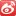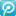2012-03-15 17:47

浏览 59222

评论

展开全文• ## Python产生随机数

万次阅读 2018-08-05 16:28:52
在数据分析中，数据的获取是第一步，numpy.random 模块提供了非常全的自动产生数据API，是学习数据分析的第一步。 总体来说，numpy.random模块...4. 生成器：种随机数种子，根据同一种子产生随机数是相同的 以下是...

在数据分析中，数据的获取是第一步，numpy.random 模块提供了非常全的自动产生数据API，是学习数据分析的第一步。
总体来说，numpy.random模块分为四个部分，对应四种功能：
1. 简单随机数： 产生简单的随机数据，可以是任何维度
2. 排列：将所给对象随机排列
3. 分布：产生指定分布的数据，如高斯分布等
4. 生成器：种随机数种子，根据同一种子产生的随机数是相同的
以下是详细内容以及代码实例：（以下代码默认已导入numpy：import numpy as np

## 1. 生成器

电脑产生随机数需要明白以下几点：
（1）随机数是由随机种子根据一定的计算方法计算出来的数值。所以，只要计算方法一定，随机种子一定，那么产生的随机数就不会变。
（2）只要用户不设置随机种子，那么在默认情况下随机种子来自系统时钟（即定时/计数器的值）
（3）随机数产生的算法与系统有关，Windows和Linux是不同的，也就是说，即便是随机种子一样，不同系统产生的随机数也不一样。
numpy.random 设置种子的方法有：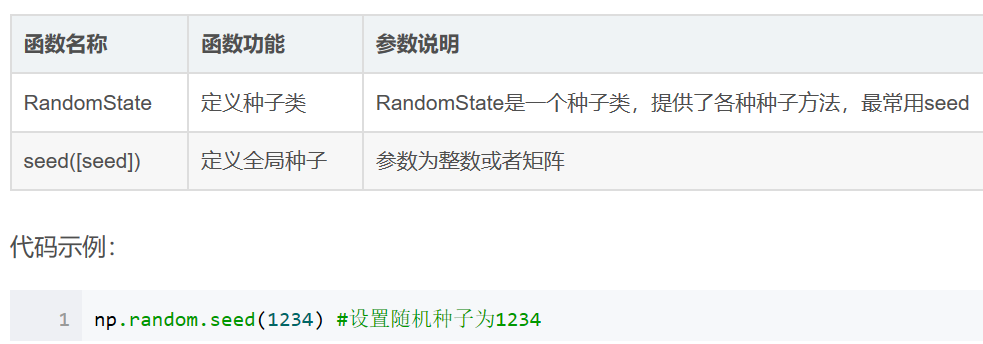np.random.RandomState(42)

2、简单随机数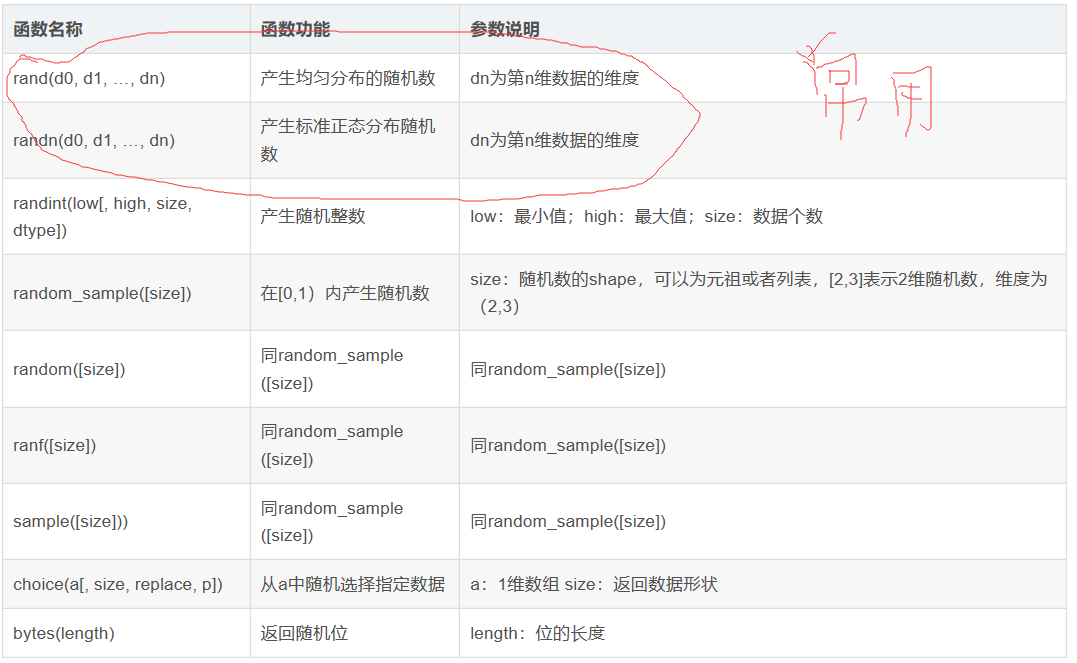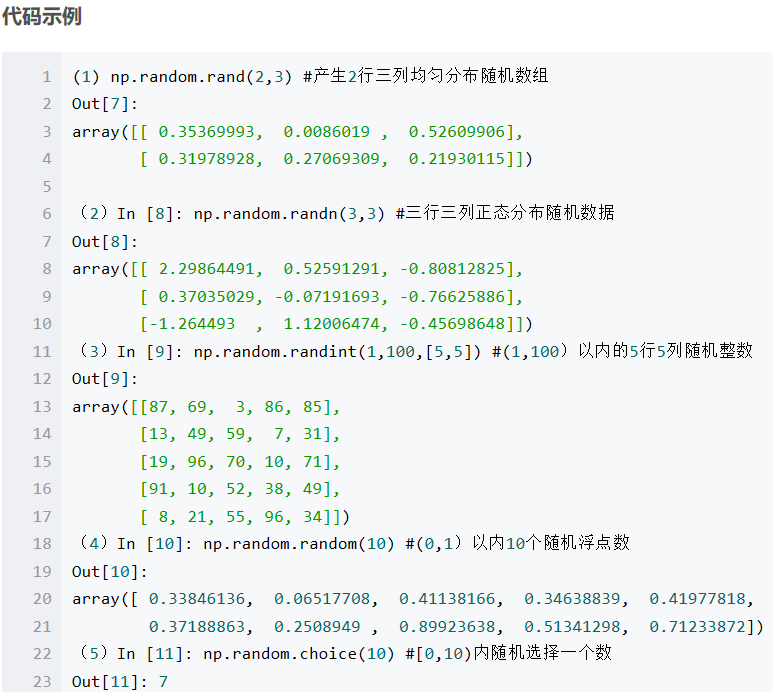例：

rng = np.random.RandomState(42) # 生成随机数时可指定一种子，目的是为了生成相同的随机数，实现代码的复现。

X = rng.randn(100, 2)

具体解读：

1、np.random.rand()

给定一个形状创建数组，并在数组中加入[0,1]之间均匀分布的随机样本。

numpy.random.rand(d0, d1, ..., dn)，产生d0 - d1 - ... - dn形状的在[0,1)上均匀分布的float型数。

2、np.random.randn()

以给定的形状创建一个数组，数组元素符合标准正态分布N(0,1)。

若要获得一般正态分布N(u,sigma2),可用 sigma*np.random.randn+u

numpy.random.randn（d0,d1,...,dn),产生d0 - d1 - ... - dn形状的标准正态分布的float型数。

3.numpy.random.randint()
用法是：numpy.random.randint(low,high=None,size=None,dtype)
生成在半开半闭区间[low,high)上离散均匀分布的整数值;若high=None，则取值区间变为[0,low)

numpy.random.randint(low, high=None, size=None, dtype='l')，产生随机整数

4.numpy.random.random_integers()
用法是： numpy.random.random_integers(low,high=None,size=None)
生成闭区间[low,high]上离散均匀分布的整数值;若high=None，则取值区间变为[1,low]

此外，若要将【a,b】区间分成N等分，也可以用此函数实现

numpy.random.random_integers(low, high=None, size=None)，在闭区间上产生随机整数；

a+(b-a)*(numpy.random.random_integers(N)-1)/(N-1)

5.numpy.random_sample()
用法是： numpy.random.random_sample(size=None)
以给定形状返回[0,1)之间的随机浮点数

6.numpy.random.choice()
用法是： numpy.random.choice(a,size=None,replace=True,p=None)
若a为数组，则从a中选取元素；若a为单个int类型数，则选取range(a)中的数
replace是bool类型，为True，则选取的元素会出现重复；反之不会出现重复
p为数组，里面存放选到每个数的可能性，即概率

7、函数原型：  numpy.random.uniform(low,high,size)

功能：从一个均匀分布[low,high)中随机采样，注意定义域是左闭右开，即包含low，不包含high.

参数介绍:

low: 采样下界，float类型，默认值为0；
high: 采样上界，float类型，默认值为1；
size: 输出样本数目，为int或元组(tuple)类型，例如，size=(m,n,k), 则输出m*n*k个样本，缺省时输出1个值

返回值：ndarray类型，其形状和参数size中描述一致。

参考：https://blog.csdn.net/u013920434/article/details/52507173

展开全文• 如果不给出将会随机给一个值且极大可能为负数 具体参考 https://www.cnblogs.com/whiteprism/p/6290814.html 3. 代码实例 导入模块 import random def FetchRandom(): '生成随机数列表并从中抓取' N1 = random....

1. 列表排序可采用两种方法

sorted(list) 直接改变list

调用list的方法 list.sort

2.

random.randint(a,b) 生成大于等于a小于等于b的整数

random.random() 生成一个在[0,1)区间上的实数

random.choice(sequence) sequence泛指list、tuple、字符串等

random.randrange(start,stop,step) step务必给出，在本次实践中，如果不给出将会随机给一个值且极大可能为负数具体参考 https://www.cnblogs.com/whiteprism/p/6290814.html

3. 代码实例

导入模块 import random

def FetchRandom():

'生成随机数列表并从中抓取'

N1 = random.randint(2,100)

N2 = random.randint(1,100)

while N2>N1:

N2 = random.randint(1,100)

alist = []

for i in range(N1):

n = random.randint(0, 2 ^ 31 - 1)

alist.append(n)

blist = []

for i in range(N2):

blist.append(random.randrange(alist,alist[N1-1],1))

#blist.append(random.choice(alist))

print sorted(blist)

print 'N1=',N1,' N2=',N2,' n=',n

4. 输出效果展开全文• Python中的random模块用于生成随机数。下面介绍一下random模块中最常用的几个函数。random.randomrandom.random()用于生成一个0到1的随机符点数: 0 <= n < 1.0random.uniformrandom.uniform的函数原型为：...
• 本文实例讲述了Python简单生成随机数的方法。分享给大家供大家参考，具体如下： 主要知识点： 随机整数： random.randint(a,b)：返回随机整数x,a<=x<=b 包含范围的随机整数 random.randrange(start,stop,[,...
• 今天学习了用python生成仿真数据的一些基本方法和技巧，写成博客和大家分享一下。本篇博客主要讲解如何从给定参数的的正态分布/均匀分布中生成随机数以及如何以给定概率从数字列表抽取某数字或从区间列表的某一区间...
• ## python生成随机数

千次阅读 2022-07-12 22:12:52python
• 通过改变第三个参数便可以更加灵活地生成想要的随机数。如当第三个参数为10时，生成的随机数便是10的倍数。下面的例子会生成指定范围内的偶数。 # -*- coding: utf-8 -*- import random print 'generate ...random 随机数
• python 产生随机数的两种途径：random库和numpy库python 开发语言 后端
• ## Python中产生随机数

万次阅读 多人点赞 2019-07-09 18:42:27
Python产生随机数： 一.Python自带的random库 1.参生n--m范围内的一个随机数: random.randint(n,m) 2.产生0到1之间的浮点数: random.random() 3.产生n---m之间的浮点数: random.uniform(1.1,5.4) 4.产生从n...
•python
• 整理下日常工作常用的python随机函数。 random.randint() random.randint(1,100) 随机产生a到b的整数，包括ab；前后ab的值，a必须小于等于b random.random() random.random() 生成一个0到1之间的随机浮点数，...python 开发语言 后端
• 记录了生成随机数的几种方式以及生成随机列表的几种方法。python 开发语言
• 在日常的生活工作和系统游戏等设计和制作时，经常会碰到产生随机数，用来解决问题，对此情况，向大家推几种可以产生随机数的方法。 1.首先需要导入random模块，使用import random导入。 random.random random....python 开发语言 后端
• 一.Python自带的random库 1.参生n–m范围内的一个随机数: random.randint(n,m) 2.产生0到1之间的浮点数: random.random() 3.产生n---m之间的浮点数: random.uniform(1.1,5.4) 4.产生从n---m间隔为k的整数: ...
• numpy 中 的random模块有多个函数用于生成不同类型的随机数，常见的有 uniform、rand、random、randint、random_interges 下面介绍一下各自的用法 1、np.random.uniform的用法 np.random.uniform(low=0.0, high...
• 之后按照固定顺序生成随机数 随机数种子对后面的结果一直有影响，在一个随机数种子后生成的随机数都会收到这个随机数种子的影响，即生成的随机数都是由这个随机数种子给的初值，按照固定顺序生成的（生成的随机数受...python
• 生成随机数使用 random 模块 random.random() 用于随机生成一个0到1的浮点数 random.randint(start,stop) 随机生成[start,stop]区间内的整数 代码示例：import randomprint (random.random())print(random.randint(2...
• Python 中用于生成随机数的模块是 random，在使用前需要 import. random.random()：生成一个 0-1 之间的随机浮点数 import random s = random.random() s random.uniform(a, b)：生成[a,b]之间的浮点数 ...python
• 前言这是俺写的第一篇关于python的博客，分享一下我所整合的python中的随机数函数，就当作是自己的一个笔记，也可以供像我一样的python入门新手小白参考学习。希望可以记录下自己的学习过程。一、random()生成一个(0...
• 这篇文章主要介绍了Python生成随机数的方法，有需要的朋友可以参考一下如果你对在Python生成随机数与random模块中最常用的几个函数的关系与不懂之处，下面的文章就是对Python生成随机数与random模块中最常用的几个......